# 8 Results

View
Selected filters:
• CCSS.Math.Content.4.G.A.3Educational Use
Rating

In this video segment from Cyberchase, a plant expert shows Bianca the patterns and symmetry found in nature.

Subject:
Life Science
Mathematics
Algebra
Material Type:
Lecture
Provider:
PBS LearningMedia
Provider Set:
PBS Learning Media: Multimedia Resources for the Classroom and Professional Development
Teachers' Domain
Author:
The William and Flora Hewlett Foundation
WNET
08/29/2008Conditional Remix & Share Permitted
CC BY-NC-SA
Rating

In this activity, learners use pattern blocks and mirrors to explore symmetry. Learners work in pairs and build mirror images of each other's designs. In doing so, learners will examine principles of symmetry and reflection.

Subject:
Physics
Material Type:
Activity/Lab
Provider:
Exploratorium
Author:
Exploratorium
Gordon and Betty Moore Foundation
National Science Foundation
The Exploratorium
12/07/2010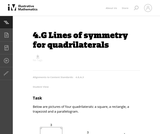Unrestricted Use
CC BY
Rating

This task provides students a chance to experiment with reflections of the plane and their impact on specific types of quadrilaterals. It is both interesting and important that these types of quadrilaterals can be distinguished by their lines of symmetry.

Subject:
Mathematics
Geometry
Material Type:
Activity/Lab
Provider:
Illustrative Mathematics
Provider Set:
Illustrative Mathematics
Author:
Illustrative Mathematics
11/11/2012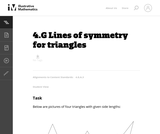Unrestricted Use
CC BY
Rating

This task is intended for instruction, providing the students with a chance to experiment with physical models of triangles, gaining spatial intuition by executing reflections.

Subject:
Mathematics
Geometry
Material Type:
Activity/Lab
Provider:
Illustrative Mathematics
Provider Set:
Illustrative Mathematics
Author:
Illustrative Mathematics
10/12/2012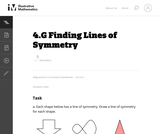Unrestricted Use
CC BY
Rating

This is a task from the Illustrative Mathematics website that is one part of a complete illustration of the standard to which it is aligned. Each task has at least one solution and some commentary that addresses important asects of the task and its potential use. Here are the first few lines of the commentary for this task: Each shape below has a line of symmetry. Draw a line of symmetry for each shape. Not every shape has an line of symmetry. Which of the four shapes belo...

Subject:
Mathematics
Material Type:
Activity/Lab
Provider:
Illustrative Mathematics
Provider Set:
Illustrative Mathematics
Author:
Illustrative Mathematics
05/14/2013Conditional Remix & Share Permitted
CC BY-NC-SA
Rating

This 20-day module introduces points, lines, line segments, rays, and angles, as well as the relationships between them. Students construct, recognize, and define these geometric objects before using their new knowledge and understanding to classify figures and solve problems. With angle measure playing a key role in their work throughout the module, students learn how to create and measure angles, as well as create and solve equations to find unknown angle measures. In these problems, where the unknown angle is represented by a letter, students explore both measuring the unknown angle with a protractor and reasoning through the solving of an equation. Through decomposition and composition activities as well as an exploration of symmetry, students recognize specific attributes present in two-dimensional figures. They further develop their understanding of these attributes as they classify two-dimensional figures based on them.

Subject:
Geometry
Material Type:
Module
Provider:
New York State Education Department
Provider Set:
EngageNY
10/20/2013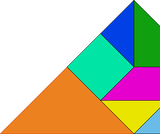Unrestricted Use
CC BY
Rating

Students learn about the history of tangrams.  They will learn about each piece in the tangram puzzle and analyze the shapes to complete geometric puzzles and mathematics problems.

Subject:
Mathematics
Geometry
Material Type:
Lesson Plan
Author:
Lynn Ann Wiscount
Vince Mariner
Erin Halovanic
07/13/2020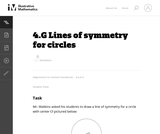Unrestricted Use
CC BY
Rating

This is an instructional task that gives students a chance to reason about lines of symmetry and discover that a circle has an an infinite number of lines of symmetry. Even though the concept of an infinite number of lines is fairly abstract, fourth graders can understand infinity in an informal way.

Subject:
Mathematics
Geometry
Material Type:
Activity/Lab
Provider:
Illustrative Mathematics
Provider Set:
Illustrative Mathematics
Author:
Illustrative Mathematics